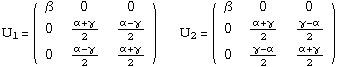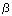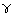## Cubic-to-Orthorhombic Transition

#### Introduction

The cubic to orthorhombic transition is described by three parameters: three stretches along three mutually perpendicular directions.

Some alloys undergoing this transition are

• Au-Cd, Cu-Al-Ni, Ti-Ta, Ti-Ni-Cu, and Ti-Pd.

There are several possible transitions between a cubic Bravais lattice and an orthorhombic Bravais lattice:

1. the simple cubic lattice can transform into either the simple or the base-centered orthorhombic lattices, and
2. the body-centered and face-centered cubic lattices are both able to transform into either the body-centered or the face-centered orthorhombic lattices.

#### Transformation Stretch Matrices

The homogeneous deformation that takes a cubic lattice into an orthorhombic lattice has gradient denoted as U which is called a transformation stretch matrix, and for the cubic-to-orthorhombic transition, there are two kinds of such matrices . The two kinds of transformation stretch matrices have

1. dilatation components exclusively (a diagonal matrix) called orthorhombic ``cubic axes'' variants or

2. both dilatation and shear components called orthorhombic ` `mixed axes'' variants.

Despite the fact that there are two possible transformation stretch matrices, experimentalists find that the second type of transformation is most commonly observed in materials which undergo a cubic-to-orthorhombic transition. In fact, we know of no materials which exhibit the ``cubic axes'' variants. Therefore, all of the calculations are with the ``mixed axes'' variants.

The transformation stretch matrices are the gradient of the deformations which take a cubic lattice into an orthorhombic lattice. There are 6 such matrices, one for each variant of the martensite phase. For the ``mixed axes'' variants, the components areThe transformation stretches,andare proportional to~ a/ao,~ b/ao, and~ c/ao, respectively, where a, b, and c are the lattice parameters of the orthorhombic lattice and ao is the lattice parameter of the cubic lattice.

#### Microstructures for the Cubic-to-Orthorhombic Transition

Here are links to various pages, where a number of different microstructures are discussed. Also contained therein is a table showing the possible microstructures for a cubic-to-orthorhombic transition.

#### References

Some references are

1. `Proposed experimental tests of a theory of fine microstructure and the two-well problem.'' by John M. Ball and Richard D. James. Philosophical Transactions of the Royal Society of London A 338, 389 (1992).

2. ``Wedge-like microstructure in martensites.'' by Kaushik Bhattacharya. Acta Metallurgica et Materialia 39, 2431 (1991).

3. ``Microstructure in a cubic to orthorhombic transition.'' by Kevin F. Hane and Thomas W. Shield. In preparation, 1998.